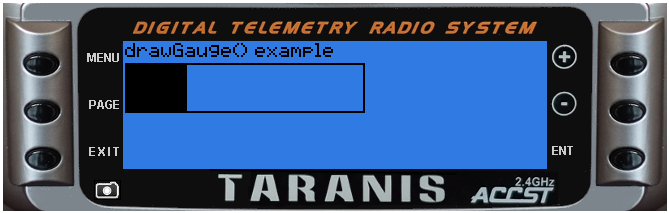## lcd.drawGauge(x, y, w, h, fill, maxfill)

Draws a simple gauge that is filled based upon fill value

@status current Introduced in 2.0.0

#### Parameters

• `x,y` (positive numbers) top left corner position

• `w` (number) width in pixels

• `h` (number) height in pixels

• `fill` (number) amount of fill to apply

• `maxfill` (number) total value of fill

• `flags` (unsigned number) drawing flags

none

### Examples

lcd/drawGauge-example

``````
local function run(event)
lcd.clear()
lcd.drawText(1,1,"drawGauge() example", 0)
lcd.drawGauge(1, 11, 120, 25, 250, 1000)
end

return{run=run}
``````